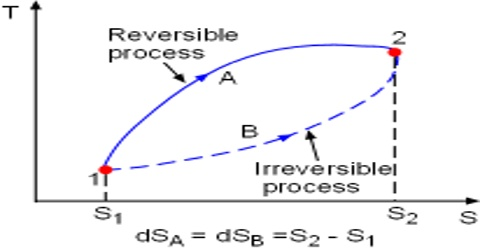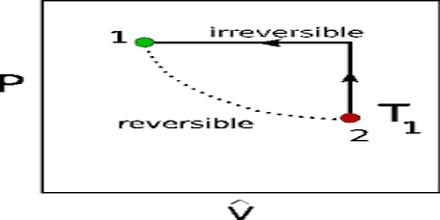# Reversible and Irreversible Processes in Thermodynamic

Reversible and irreversible processes in Thermodynamic

When the state of a system is changed by changing any of the variables on which it is dependent, the operation is called a thermodynamic process. Different types of thermodynamic process are:

(i) Reversible and irreversible,

(iii) Isochoric and isobaric and

(iv) Cyclic process

Here briefly focus on Reversible and irreversible processes;

Reversible and irreversible processes

One of the most important concepts in thermodynamics is that of reversibility. A thermodynamically reversible process is defined as, “A process that takes place infinitesimally slowly in infinite number of small steps and requires infinite time to reach the final state. The process takes place in such a way that each step is at equilibrium with the preceding step. The reversible process can be made to go in the reverse direction by infinitesimal change in the state of the system”.

In contrast, “the irreversible process takes place at finite time and the system is at equilibrium only at the initial and final state. Irreversible processes are real and can be performed in real world”.

One of the major requirements for carrying out a reversible process involving gases is the availability of a weightless and frictionless piston which is not feasible in practice. In order to better understand the concept of reversible and irreversible processes two examples are given.Example 1: Let us consider that a liquid is placed in a cylinder fitted with a weightless and frictionless piston. The cylinder with the liquid is placed in a large reservoir maintained at the boiling temperature of the liquid. The vapour pressure of the liquid will be equal to the atmospheric pressure and the system will be in equilibrium at this temperature. If the pressure on the piston is now increased by an infinitesimally small amount dP, small quantity of the vapour condenses and the heat given up will be dissipated in the reservoir so that the temperature does not rise. As the increase in pressure is infinitesimally small the condensation will take place very slowly and the system will be in equilibrium at all times. If the temperature of the reservoir is raised by an infinitesimal amount dT, the vapour pressure of the liquid will be slightly greater and the piston will be raised by a small amount. More liquid will evaporate and the pressure of the vapour in the cylinder is maintained constant. The temperature in the cylinder tends to decrease due to expansion of the vapour but is maintained constant by flow of heat from the reservoir. On the other hand, if the external pressure is decreased by a very small amount dP, the liquid sill evaporate at a slow rate.

The system will remain at equilibrium and the process will be reversible. If, however, the pressure change is made appreciable, sudden evaporation or condensation will take place rapidly and since the system is not at equilibrium during the process, it is irreversible.

Example 2: Another example of a reversible process is an electrolytic cell. Let consider a Daniel cell. If the electrodes of the cell are connected externally, current will flow in one direction. If an external sources of e.m.f is connected to the Daniel cell such that the e.m.f. of the external is in opposite direction and is slightly higher than that of the Daniel cell, current in the latter will flow in the opposite direction, i.e., from the external cell to the Daniel cell. If the e.m.f. of the external source is slightly smaller, current will flow from the Daniel cell into the external source. In this sense the process is reversible as it may be reversed by small changes one way or the other of the voltage.

A reversible process is a hypothetical one since infinite number of steps is required to complete the process. Infinitesimally small changes are impossible to carry out in practice. All processes that occur in nature are more or less irreversible. It should, however, be mentioned that reversible processes yield the maximum work.

Examples of irreversible processes are abundant in Nature, e.g. flowing of rivers downstream, blooming of flowers in the gardens or evolution of hydrogen when a piece of zinc is placed in acid etc.